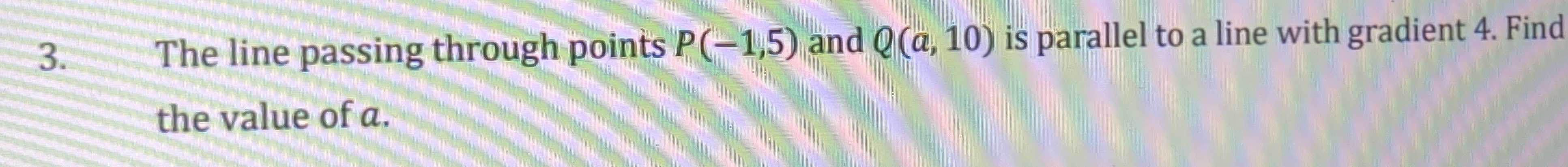### Still have math questions?3. The line passing through points $$P ( - 1,5 )$$ and $$Q ( a , 10 )$$ is parallel to a line with gradient 4. Find the value of $$a$$ .